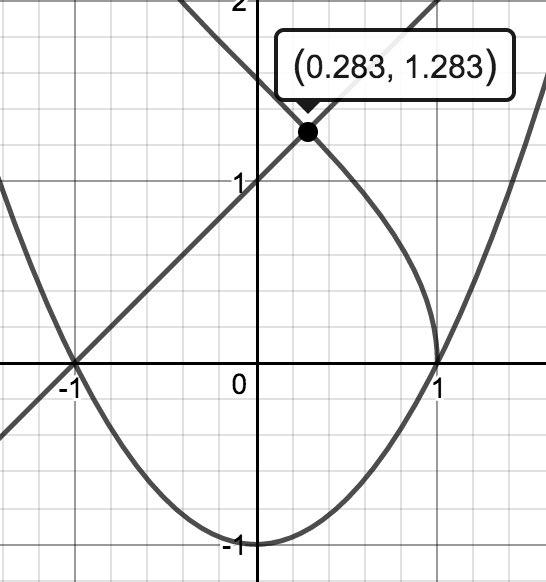### Home > CALC3RD > Chapter Ch9 > Lesson 9.4.1 > Problem9-111

9-111.

Calculate the area of the region which includes the origin and is bounded by the curves $f(x) = x + 1$, $g(x) = x^2 - 1$, and $h(x) = \cos^{–1}(x)$.

Graph the curves and determine the points of intersection.$\int_{-1}^{0.283}\big(f(x)-g(x)\big)dx+\int_{0.283}^1\big(h(x)-g(x)\big)dx$

For the second integral, use integration by parts.
Let $f = \cos^{–1}(x)$ and $dg = 1dx$.